International
Tables for
Crystallography
Volume F
Crystallography of biological molecules
Edited by M. G. Rossmann and E. Arnold

International Tables for Crystallography (2006). Vol. F, ch. 8.1, pp. 162-164   | 1 | 2 |

Section 8.1.7. SR monochromatic and Laue diffraction geometry

J. R. Helliwella*

aDepartment of Chemistry, University of Manchester, M13 9PL, England
Correspondence e-mail: john.helliwell@man.ac.uk

8.1.7. SR monochromatic and Laue diffraction geometry

| top | pdf |

In the utilization of SR, both Laue and monochromatic modes are important for data collection. The unique geometric and spectral properties of SR render the treatment of diffraction geometry different from that for a conventional X-ray source.

8.1.7.1. Laue geometry: sources, optics, sample reflection bandwidth and spot size

| top | pdf |

Laue geometry involves the use of the polychromatic SR spectrum as transmitted through the beryllium window that is used to separate the apparatus from the machine vacuum. There is useful intensity down to a wavelength minimum of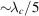, whereis the critical wavelength of the magnet source. The maximum wavelength is typically ≥3 Å; however, if the crystal is mounted in a capillary, then the glass absorbs the wavelengths beyond ~2.5 Å.

The bandwidth can be limited somewhat under special circumstances. A reflecting mirror at grazing incidence can be used for two purposes. First, the minimum wavelength in the beam can be sharply defined to aid the accurate definition of the Laue spot multiplicity. Second, the mirror can be used to focus the beam at the sample. The maximum-wavelength limit can be truncated by use of aluminium absorbers of varying thickness or a transmission mirror (Lairson & Bilderback, 1982; Cassetta et al., 1993).

The measured intensity of individual Laue diffraction spots depends on the wavelength at which they are stimulated. The problem of wavelength normalization is treated by a variety of methods. These include:

 (i) use of a monochromatic reference data set; (ii) use of symmetry equivalents in the Laue data set recorded at different wavelengths; (iii) calibration with a standard sample, such as a silicon crystal.

Each of these methods produces a λ curve' describing the relative strength of spots measured at various wavelengths. The methods rely on the incident spectrum being smooth and stable with time. The bromine and silver K absorption edges, in AgBr photographic film, lead to discontinuities in the λ-curve. Hence, the λ-curve is usually split up into wavelength regions, for exampleto 0.49 Å, 0.49 to 0.92 Å, and 0.92 Å to. Other detector types have different discontinuities, depending on the material making up the X-ray absorbing medium. Most Laue diffraction data now recorded on CCDs or IPs. The greater sensitivity of these detectors (expressed as the DQE), especially for weak signals, has greatly increased the number of Laue exposures recordable per crystal. Thus, multiplet deconvolution procedures, based on the recording of reflections stimulated at different wavelengths and with different relative intensities, have become possible (Campbell & Hao, 1993; Ren & Moffat, 1995b). Data quality and completeness have improved considerably.

The production and use of narrow-bandpass beams, e.g.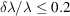, may be of interest for enhancing the signal-to-noise ratio. Such bandwidths can be produced by a combination of a reflection mirror used in tandem with a transmission mirror. Alternatively, an X-ray undulator of 10–100 periods should ideally yield a bandwidth behind a pinhole of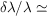0.1–0.01. In these cases, wavelength normalization is more difficult, because the actual spectrum over which a reflection is integrated is rapidly varying in intensity; nevertheless, high-order Chebychev polynomials are successful (Ren & Moffat, 1995a).

The spot bandwidth is determined by the mosaic spread and horizontal beam divergence (since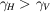) as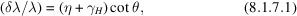where η is the sample mosaic spread, assumed to be isotropic,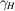is the horizontal cross-fire angle, which in the absence of focusing is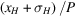, where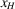is the horizontal sample size,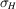is the horizontal source size and P is the sample to the tangent-point distance. This is similar for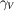in the vertical direction. Generally, at SR sources,is greater than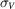. When a focusing-mirror element is used,and/orare convergence angles determined by the focusing distances and the mirror aperture.

The size and shape of the diffraction spots vary across the detector image plane. The radial spot length is given by convolution of Gaussians as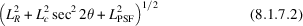and tangentially by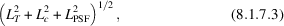whereis the size of the X-ray beam (assumed to be circular) at the sample,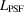is the detector point spread factor,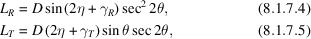and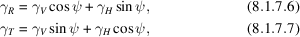where ψ is the angle between the vertical direction and the radius vector to the spot (see Andrews et al., 1987). For a crystal that is not too mosaic, the spot size is dominated byand. For a mosaic or radiation-damaged crystal, the main effect is a radial streaking arising from η, the sample mosaic spread.

8.1.7.2. Monochromatic SR beams: optical configurations and sample rocking width

| top | pdf |

A wide variety of perfect-crystal monochromator configurations are possible and have been reviewed by various authors (Hart, 1971; Bonse et al., 1976; Hastings, 1977; Kohra et al., 1978). Since the reflectivity of perfect silicon and germanium is effectively 100%, multiple-reflection monochromators are feasible and permit the tailoring of the shape of the monochromator resolution function, harmonic rejection and manipulation of the polarization state of the beam. Two basic designs are in common use. These are the bent single-crystal monochromator of triangular shape (Fig. 8.1.4.1a) and the double-crystal monochromator (Fig. 8.1.4.1b).

8.1.7.2.1. Curved single-crystal monochromator

| top | pdf |

In the case of the single-crystal monchromator, the actual curvature employed is very important in the diffraction geometry. For a point source and a flat monochromator crystal, there is a gradual change in the photon wavelength selected from the white beam as the length of the monochromator is traversed (Fig. 8.1.7.1a). For a point source and a curved monochromator crystal, one specific curvature can compensate for this variation in incidence angle (Fig. 8.1.7.1b). The reflected spectral bandwidth is then at a minimum; this setting is known as the Guinier position'. If the curvature of the monochromator crystal is increased further, a range of photon wavelengths,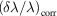, is selected along its length so that the rays converging towards the focus have a correlation of photon wavelength and direction (Fig. 8.1.7.1c). The effect of a finite source is to cause a change in incidence angle at the monochromator crystal, so that at the focus there is a photon-wavelength gradient across the width of the focus (for all curvatures) (Fig. 8.1.7.1d). The use of a slit in the focal plane is akin to placing a slit at the tangent point to limit the source size.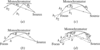Figure 8.1.7.1 | top | pdf |Single-crystal monochromator illuminated by SR. (a) Flat crystal. (b) Guinier setting. (c) Overbent crystal. (d) Effect of source size (shown at the Guinier setting for clarity). From Helliwell (1984). Reproduced with the permission of the Institute of Physics.

8.1.7.2.2. Double-crystal monochromator

| top | pdf |

The double-crystal monochromator with two parallel or nearly parallel perfect crystals of germanium or silicon is a common configuration. The advantage of this is that the outgoing monochromatic beam is parallel to the incoming beam, although it is slightly displaced vertically by an amount, where d is the perpendicular distance between the crystals and θ is the monochromator Bragg angle (unless the second crystal is unconnected to the first, in which case it can be translated as well to compensate for that). The monochromator can be rapidly tuned, since the diffractometer or camera need not be re-aligned significantly in a scan across an absorption edge. Since the rocking width of the fundamental is broader than the harmonic reflections, the strict parallelism of the pair of the crystal planes can be relaxed or detuned', so that the harmonic can be rejected with little loss of the fundamental intensity. The spectral spread in the reflected monochromatic beam is determined by the source divergence accepted by the monochromator, the angular size of the source and the monochromator rocking width (see Fig. 8.1.7.2). The double-crystal monochromator is often used with a toroidal focusing mirror; the functions of monochromatization are then separated from the focusing (Hastings et al., 1978).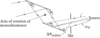Figure 8.1.7.2 | top | pdf |Double-crystal monochromator illuminated by SR. The contributions of the source divergence, αV [less than or equal to γ−1, equation (8.1.2.4), depending on the monochromator vertical entrance slit aperture; see also Colapietro et al., 1992], and angular source size,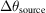, to the range of energies reflected by the monochromator are shown. From Helliwell (1984). Reproduced with the permission of the Institute of Physics.

8.1.7.2.3. Crystal sample rocking width

| top | pdf |

The rocking width of a reflection depends on the horizontal and vertical beam divergence or convergence (after due account for collimation is taken),and, the spectral spreads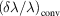and, and the mosaic spread, η. We assume that the mosaic spread η is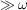, the angular broadening of a reciprocal-lattice point (relp) due to a finite sample. In the case of synchrotron radiation,andare usually widely asymmetric. On a conventional source, usually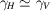. Two types of spectral spread occur with synchrotron (and neutron) sources. The termis the spread that is passed down each incident ray in a divergent or convergent incident beam; the subscript refers to the conventional source type. This is because it is similar to the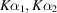line widths and separation. At the synchrotron, this component also exists and arises from the monochromator rocking width and finite-source-size effects. The termis special to the synchrotron or neutron case. The subscript corr' refers to the fact that the ray direction can be correlated with the photon or neutron wavelength. In this most general case, and for one example of aarising from the horizontal ray direction correlation with photon energy and the case of a horizontal rotation axis, the rocking width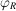of an individual reflection is given by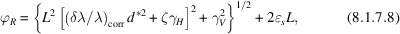where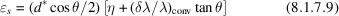and L is the Lorentz factor,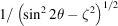.

The Guinier setting of an instrument (curved crystal monochromator case, Fig. 8.1.7.1b) gives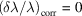. The equation forthen reduces to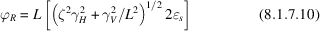(from Greenhough & Helliwell, 1982). For example, for,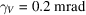(0.01°),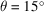,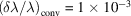and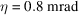(0.05°), then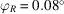. Butincreases as ζ increases [see Greenhough & Helliwell (1982), Table 5]. In the rotation/oscillation method as applied to protein and virus crystals, a small angular range is used per exposure. For example, the maximum rotation range per image,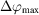, may be 1.5° for a protein and 0.4° or so for a virus. Many reflections will be only partially stimulated over the exposure. It is important, especially in the virus case, to predict the degree of penetration of the relp through the Ewald sphere. This is done by analysing the interaction of a spherical volume for a given relp with the Ewald sphere. The radius of this volume is given by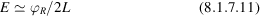(Greenhough & Helliwell, 1982).

In Fig. 8.1.7.3, the relevant parameters are shown. The diagram shows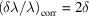in a plane, usually horizontal with a perpendicular (vertical) rotation axis, whereas the formula forabove is for a horizontal axis. This is purely for didactic reasons since the interrelationship of the components is then much clearer.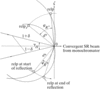Figure 8.1.7.3 | top | pdf |The rocking width of an individual reflection for the case of Fig. 8.1.7.1(c)and a vertical rotation axis. From Greenhough & Helliwell (1982).

References

Andrews, S. J., Hails, J. E., Harding, M. M. & Cruickshank, D. W. J. (1987). The mosaic spread of very small crystals deduced from Laue diffraction patterns. Acta Cryst. A43, 70–73.Google Scholar
Bonse, U., Materlik, G. & Schröder, W. (1976). Perfect-crystal monochromators for synchrotron X-radiation. J. Appl. Cryst. 9, 223–230.Google Scholar
Campbell, J. W. & Hao, Q. (1993). Evaluation of reflection intensities for the components of multiple Laue diffraction spots. II. Using the wavelength-normalization curve. Acta Cryst. A49, 889–893.Google Scholar
Cassetta, A., Deacon, A., Emmerich, C., Habash, J., Helliwell, J. R., McSweeney, S., Snell, E., Thompson, A. W. & Weisgerber, S. (1993). The emergence of the synchrotron Laue method for rapid data collection from protein crystals. Proc. R. Soc. London Ser. A, 442, 177–192.Google Scholar
Greenhough, T. J. & Helliwell, J. R. (1982). Oscillation camera data processing: reflecting range and prediction of partiality. II. Monochromatised synchrotron X-radiation from a singly bent triangular monochromator. J. Appl. Cryst. 15, 493–508.Google Scholar
Hart, M. (1971). Bragg reflection X-ray optics. Rep. Prog. Phys. 34, 435–490.Google Scholar
Hastings, J. B. (1977). X-ray optics and monochromators for synchrotron radiation. J. Appl. Phys. 48, 1576–1584.Google Scholar
Hastings, J. B., Kincaid, B. M. & Eisenberger, P. (1978). A separated function focusing monochromator system for synchrotron radiation. Nucl. Instrum. Methods, 152, 167–171.Google Scholar
Kohra, K., Ando, M., Matsushita, T. & Hashizume, H. (1978). Design of high resolution X-ray optical system using dynamical diffraction for synchrotron radiation. Nucl. Instrum. Methods, 152, 161–166.Google Scholar
Lairson, B. M. & Bilderback, D. H. (1982). Transmission X-ray mirror – a new optical element. Nucl. Instrum. Methods, 195, 79–83.Google Scholar
Ren, Z. & Moffat, K. (1995a). Quantitative analysis of synchrotron Laue diffraction patterns in macromolecular crystallography. J. Appl. Cryst. 28, 461–481.Google Scholar
Ren, Z. & Moffat, K. (1995b). Deconvolution of energy overlaps in Laue diffraction. J. Appl. Cryst. 28, 482–493.Google Scholar